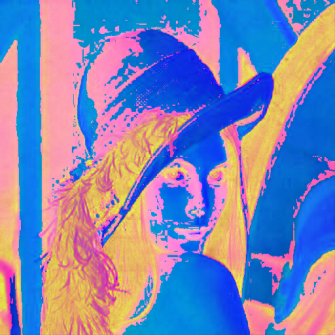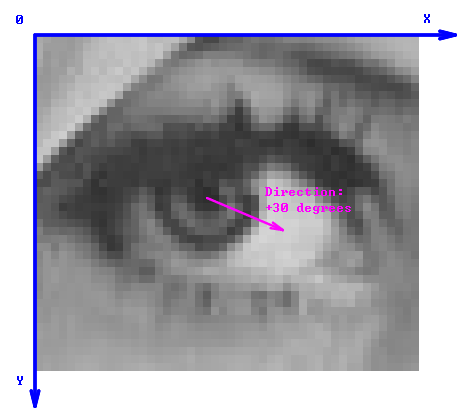# Image

### Description

The Image data type stores information about dimensions, pixel format and raw pixel values. The list of possible formats is similar to the one from the OpenCV library – there can be from 1 to 4 channels and 6 possible primitive types. For maximum flexibility handling of specific color spaces is left to appropriate filters.A single-channel image.A 3-channel RGB image.A 3-channel HSV image displayed as RGB.

The Image structure contains the following fields:

• Width : Integer – number of pixel columns
• Height : Integer – number of pixel rows
• Pitch : Integer – byte-distance between consecutive rows
• Type : PlainType – type of pixel components
• Depth : Integer – number of channels

Possible pixel types are:

• SInt8 – integer number from -128 to 127
• UInt8 – integer number from 0 to 255
• SInt16 – integer number from -32768 to 32767
• UInt16 – integer number from 0 to 65535
• SInt32 – Integer number from -2147483648 to 2147483647
• Real – Real number

Note that the vast majority of images use the UInt8 type.

## Geometrical Coordinates

If w and h are the image dimensions, then all real-valued coordinates within the image are included in the ranges from 0.0 to w and from 0.0 to h. The top left pixel of the image covers the square area with the top-left corner at (0.0, 0.0) and the bottom-right corner at (1.0, 1.0). The X axis is directed to the right, the Y axis downwards.

Angles are denoted in degrees. For directions, the angle 0 denotes the direction of the X axis, i.e. the direction from left to right. The angles increase in the clock-wise manner.## Remarks

• A region of interest, if required, is supposed to be provided as a separate object.
• The channels of an image are interleaved.
• Maximum image dimensions are 65536 x 65536.
• All pixel rows are guaranteed to start at a 16 bytes-aligned memory address.